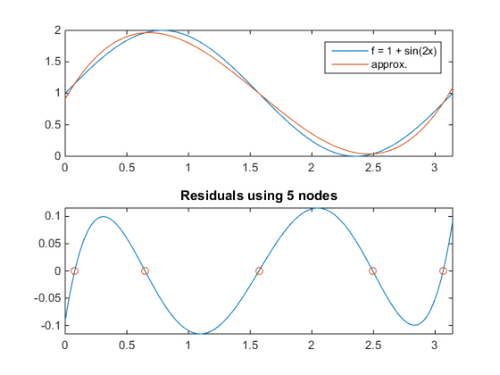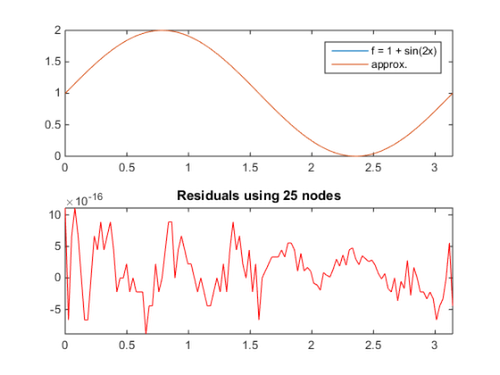# DEMO: basisChebyshev

This script provides a few examples on using the basis class.

Last updated: October 4, 2014.

## EXAMPLE 1: Using basisChebyshev to interpolate a 1-D function with a Chebyshev basis

PROBLEM: Interpolate the function y = f(x) = 1 + sin(2*x) on the domain [0,pi], using 5 Gaussian nodes.

• First, create the function f
```f = @(x) 1 + sin(2*x);
```
• and the Chebyshev basis B. If the type of nodes is unspecified, Gaussian is computed by default
```B = basisChebyshev(5,0,pi)
```
```Chebyshev Basis of one dimension with gaussian nodes

Variable           # of nodes       Interval
V0                         5        [  0.00,   3.14]

```

## Interpolation matrix and nodes

• Obtain the interpolation matrix Phi, evaluated at the basis nodes.
```Phi = B.Interpolation
```
```Phi =

1.0000   -0.9511    0.8090   -0.5878    0.3090
1.0000   -0.5878   -0.3090    0.9511   -0.8090
1.0000         0   -1.0000         0    1.0000
1.0000    0.5878   -0.3090   -0.9511   -0.8090
1.0000    0.9511    0.8090    0.5878    0.3090

```
• The basis nodes are:
```xnodes = B.nodes
```
```xnodes =

0.0769
0.6475
1.5708
2.4941
3.0647

```

## Fitting a function

• To set the interpolation coefficients c:
```c = Phi\f(xnodes)
```

or simply

```c = B.Interpolation\f(B.nodes)
```
```c =

1.0000
-0.5690
-0.0000
0.6601
0.0000

```

Next plot the function f and its approximation. To evaluate the function defined by the basis B and coefficients c at values xx we use the Interpolate method:

```y_approx = B.Interpolate(c,xx)
```

We also plot the residuals, showing the residuals at the interpolating nodes (zero by construction)

```xx = linspace(B.a,B.b,121)';
figure
subplot(2,1,1), plot(xx,[f(xx), B.Interpolate(c,xx)])
axis tight
legend('f = 1 + sin(2x)','approx.')

subplot(2,1,2), plot(xx, B.Interpolate(c,xx) - f(xx))
title('Residuals using 5 nodes'), axis tight
hold on, plot(B.nodes,f(B.nodes) - B.Interpolate(c),'o')
```## Adjusting the number of nodes

To increase accuracy, we increase the number of nodes in B to 25.

```B.n = 25;
c2 = B.Interpolation\f(B.nodes);
figure
subplot(2,1,1), plot(xx,[f(xx), B.Interpolate(c2,xx)])
axis tight
legend('f = 1 + sin(2x)','approx.')

subplot(2,1,2), plot(xx, B.Interpolate(c2,xx) - f(xx),'r')
title('Residuals using 25 nodes'), axis tight
```